Test: Normal Forms- 1

# Test: Normal Forms- 1 - Computer Science Engineering (CSE)

Test Description

## 20 Questions MCQ Test GATE Computer Science Engineering(CSE) 2024 Mock Test Series - Test: Normal Forms- 1

Test: Normal Forms- 1 for Computer Science Engineering (CSE) 2023 is part of GATE Computer Science Engineering(CSE) 2024 Mock Test Series preparation. The Test: Normal Forms- 1 questions and answers have been prepared according to the Computer Science Engineering (CSE) exam syllabus.The Test: Normal Forms- 1 MCQs are made for Computer Science Engineering (CSE) 2023 Exam. Find important definitions, questions, notes, meanings, examples, exercises, MCQs and online tests for Test: Normal Forms- 1 below.
Solutions of Test: Normal Forms- 1 questions in English are available as part of our GATE Computer Science Engineering(CSE) 2024 Mock Test Series for Computer Science Engineering (CSE) & Test: Normal Forms- 1 solutions in Hindi for GATE Computer Science Engineering(CSE) 2024 Mock Test Series course. Download more important topics, notes, lectures and mock test series for Computer Science Engineering (CSE) Exam by signing up for free. Attempt Test: Normal Forms- 1 | 20 questions in 60 minutes | Mock test for Computer Science Engineering (CSE) preparation | Free important questions MCQ to study GATE Computer Science Engineering(CSE) 2024 Mock Test Series for Computer Science Engineering (CSE) Exam | Download free PDF with solutions
 1 Crore+ students have signed up on EduRev. Have you?
Test: Normal Forms- 1 - Question 1

### Relation R is decomposed using a set of functional dependencies, F and relation S is decomposed using another set of functional dependencies G. One decomposition is definitely BCNF, the other is definitely 3NF, but it is not known which is which. To make a guaranteed identification, which one of the following tests should be used on the decompositions? (Assume that the closures of F and G are available).

Detailed Solution for Test: Normal Forms- 1 - Question 1

Answer is (C) since to identify BCNF we need BCNF definition. One relation which satisfies will be in BCNF and other will be in 3NF. 1st is wrong because dependency may be preserved by both 3NF and BCNF. 2nd is wrong Because both 3NF and BCNF decomposition can be lossless. 4th is wrong because 3NF and BCNF both are in 3NF also.

Test: Normal Forms- 1 - Question 2

### From the following instance of a relation scheme R (A, B, C), we can conclude that :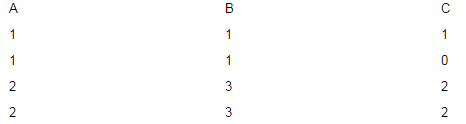Detailed Solution for Test: Normal Forms- 1 - Question 2

Generally Normalization is done on the schema itself. From the relational instance given,we may strike out FD s that do not hold. e.g.B does not functionally determine C(This is true). But we cannot say that A functionally determines B for the entire relation itself. This is because that, A->B holds for this instance, but in future there might be some tuples added to the instance that may violate A->B. So overall on the relation we cannot conclude that A->B, from the relational instance which is just a subset of an entire relation.

Test: Normal Forms- 1 - Question 3

### Consider a schema R(A,B,C,D) and functional dependencies A->B and C->D. Then the decomposition of R into R1(AB) and R2(CD) is

Detailed Solution for Test: Normal Forms- 1 - Question 3

Dependency Preserving Decomposition:
Decomposition of R into R1 and R2 is a dependency preserving decomposition if closure of functional dependencies after decomposition is same as closure of of FDs before decomposition.
A simple way is to just check whether we can derive all the original FDs from the FDs present after decomposition.

In the above question R(A, B, C, D) is decomposed into R1 (A, B) and R2(C, D) and there are only two FDs A -> B and C -> D. So, the decomposition is dependency preserving

Lossless-Join Decomposition:
Decomposition of R into R1 and R2 is a lossless-join decomposition if at least one of the following functional dependencies are in F+ (Closure of functional dependencies)

R1 ∩ R2 → R1
OR
R1 ∩ R2 → R2

In the above question R(A, B, C, D) is decomposed into R1 (A, B) and R2(C, D), and R1 ∩ R2 is empty. So, the decomposition is not lossless.

Test: Normal Forms- 1 - Question 4

Suppose the adjacency relation of vertices in a graph is represented in a table Adj(X,Y). Which of the following queries cannot be expressed by a relational algebra expression of constant length?

Detailed Solution for Test: Normal Forms- 1 - Question 4

(A) This is simple query as we need to find (X, Y) for a given X. (B) This is also simple as need to find (X, X) (C) :-> Cycle < 3 . Means cycle of length 1 & 2. Cycle of length 1 is easy., Same as self loop. Cycle of length 2 is is also not too hard to compute. Though it'll be little complex, will need to do like (X,Y) & (Y, X ) both present & X != Y,. We can do this with constant RA query. (D) :-> This is most hard part. Here we need to find closure of vertices. This will need kind of loop. If the graph is like skewed tree, our query must loop for O(N) Times. We can't do with constant length query here. Answer is :-> D

Test: Normal Forms- 1 - Question 5

Let r and s be two relations over the relation schemes R and S respectively, and let A be an attribute in R. then the relational algebra expression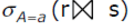is always equal to

Detailed Solution for Test: Normal Forms- 1 - Question 5

The above expression evaluates A = a for tables r and s Option A : is only displaying attributes of table r on the select condition Option B : is only displaying attributes of table rOption C: evaluates A = a by joining tables r and s  efficiently , thus correct Therefore, Answer C

Test: Normal Forms- 1 - Question 6

R(A,B,C,D) is a relation. Which of the following does not have a lossless join, dependency preserving BCNF decomposition?

Detailed Solution for Test: Normal Forms- 1 - Question 6

Background :

• Lossless-Join Decomposition:
Decomposition of R into R1 and R2 is a lossless-join decomposition if at least one of the following functional dependencies are in F+ (Closure of functional dependencies)

R1 ∩ R2 → R1
OR
R1 ∩ R2 → R2

• dependency preserving :
Decomposition of R into R1 and R2 is a dependency preserving decomposition if closure of functional dependencies after decomposition is same as closure of of FDs before decomposition.
A simple way is to just check whether we can derive all the original FDs from the FDs present after decomposition.

Question : We know that for lossless decomposition common attribute should be candidate key in one of the relation. A) A->B, B->CD R1(AB) and R2(BCD) B is the key of second and hence decomposition is lossless. B) A->B, B->C, C->D R1(AB) , R2(BC), R3(CD) B is the key of second and C is the key of third, hence lossless. C) AB->C, C->AD R1(ABC), R2(CD) C is key of second, but C->A violates BCNF condition in ABC as C is not a key. We cannot decompose ABC further as AB->C dependency would be lost.D) A ->BCD Already in BCNF. Therefore, Option C AB->C, C->AD is the answer.

Test: Normal Forms- 1 - Question 7

Which of the following relational calculus expressions is not safe?

Detailed Solution for Test: Normal Forms- 1 - Question 7

A tuple relational calculus expression may at times generate an infinite relation. It may also contain values that do not even appear in the database. Such expressions are said to be unsafe. safe tuple relational calculus expression is the one which surely generates finite results. To pose a restriction over the unsafety of expressions in tuple relational calculus there is a concept of domain of a tuple relational formula denoted by dom (P) is the set of values referenced by P i.e. values there in P or values in tuple of a relation mentioned in P. Eg: The expression {t | ¬ (t € R)} is not safe because there are infinitely many tuples that do not occur in R relation . In the above question Options (A), (B) and option (D) produce finite set of tuples as each gives out tuples restricted from a particular relation and hence are safe. Option (C) produces infinite number of tuples as it generates all the tuples not in R1 i.e. it can have tuples from any other relation other than R1.Hence it is not safe.

Test: Normal Forms- 1 - Question 8

Consider a relation geq which represents “greater than or equal to”, that is, (x,y) ∈ geq only if y >= x.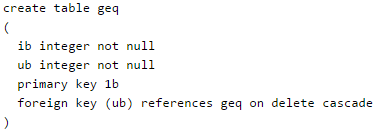Q. Which of the following is possible if a tuple (x,y) is deleted?

Detailed Solution for Test: Normal Forms- 1 - Question 8

In the above question, the relation schema is (lb , ub), where lb is the primary key, and ub is the foreign key which is referencing the primary key of its own relation. Hence the table geq is both the master (which has the referenced key) as well as the child table (which has the referencing key). The table has two constraint, one is that if there is a tuple (x, y), then y is greater than or equal to x, And the other is referential integrity constraint, which is on-cascade-delete on the foreign key. On-cascade-delete says, that "When the referenced row is deleted from the other table (master table), then delete also from the child table".

Suppose the instance in the given relation is the following: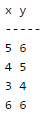Now if we delete tuple (5,6) then tuple (4,5) should also be deleted (as 5 in the tuple (4, 5) was referencing to 5 in the tuple(5,6) which no longer exist, hence the referencing tuple should also be deleted), and as (4,5) got deleted hence tuple (3,4) should also be deleted for the same reason. Therefore in total 3 rows have to be deleted if tuple (5,6) is deleted. Now from the above instance we can say that if (x,y), i.e. ( 5,6 ) gets deleted then a tuple (z, w) i.e, (3, 4) is also deleted. And we can see here that w < x. Hence option C.

Test: Normal Forms- 1 - Question 9

Given the relations

employee (name, salary, deptno) and department (deptno, deptname, address)

Q. Which of the following queries cannot be expressed using the basic relational algebra operations (U, -, x,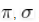, p)?

Test: Normal Forms- 1 - Question 10

Given the following relation instance.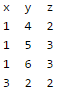Which of the following functional dependencies are satisfied by the instance?

Test: Normal Forms- 1 - Question 11

Consider an Entity-Relationship (ER) model in which entity sets E1 and E2 are connected by an m : n relationship R12, E1 and E3 are connected by a 1 : n (1 on the side of E1 and n on the side of E3) relationship R13. E1 has two single-valued attributes a11 and a12 of which a11 is the key attribute. E2 has two single-valued attributes a21 and a22 is the key attribute. E3 has two single-valued attributes a31 and a32 of which a31 is the key attribute. The relationships do not have any attributes. If a relational model is derived from the above ER model, then the minimum number of relations that would be generated if all the relations are in 3NF is ___________.

Detailed Solution for Test: Normal Forms- 1 - Question 11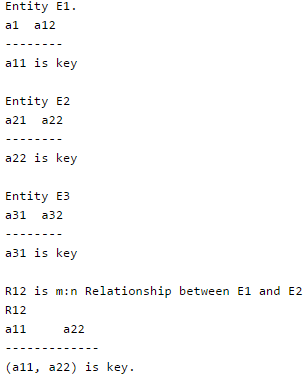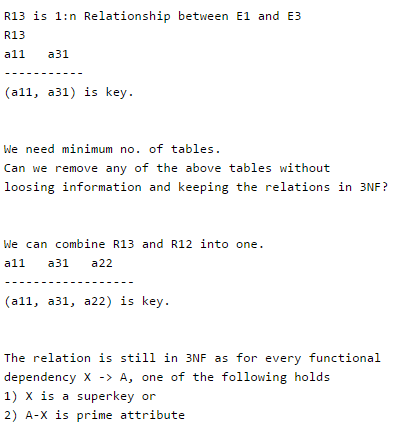Test: Normal Forms- 1 - Question 12

Consider the relation X(P, Q, R, S, T, U) with the following set of functional dependencies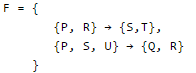Q. Which of the following is the trivial functional dependency in F+ is closure of F?

Detailed Solution for Test: Normal Forms- 1 - Question 12

A functional dependency X -> Y is trivial if Y is a subset of X.

Test: Normal Forms- 1 - Question 13

Consider the following entity relationship diagram (ERD), where two entities E1 and E2 have a relation R of cardinality 1 : m.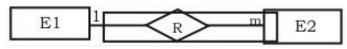The attributes of E1 are A11, A12 and A13 where A11 is the key attribute. The attributes of E2 are A21, A22 and A23 where A21 is the key attribute and A23 is a multi-valued attribute. Relation R does not have any attribute. A relational database containing minimum number of tables with each table satisfying the requirements of the third normal form (3NF) is designed from the above ERD. The number of tables in the database is

Detailed Solution for Test: Normal Forms- 1 - Question 13

Step 1: 1NF T1: A11, A12, A13 T2: A11, A21, A22, A23  //because A23 is multivalued ,it has to be included in Key attribute Step 2: 2NF // A23 is Multivalued attribute and not allowed in 2NF therefore new tables are: T1: A11, A12, A13 T2: A11, A21, A22 T3: A21, A23 Step 3: 3NF // There is no transitive functional dependency in all tables , So in 3NFTherefore answer is B

Test: Normal Forms- 1 - Question 14

A relational database contains two tables student and department in which student table has columns roll_no, name and dept_id and department table has columns dept_id and dept_name. The following insert statements were executed successfully to populate the empty tables:

Insert into department values (1, 'Mathematics')

Insert into department values (2, 'Physics')
Insert into student values (l, 'Navin', 1)
Insert into student values (2, 'Mukesh', 2)
Insert into student values (3, 'Gita', 1)

Q. How many rows and columns will be retrieved by the following SQL statement?

Select * from student, department

Detailed Solution for Test: Normal Forms- 1 - Question 14

Simple,Cartesian product of two tables will result Rows = 3*2=6 Columns= 3+2=5 So Answer is D

Test: Normal Forms- 1 - Question 15

Consider the entities 'hotel room', and 'person' with a many to many relationship 'lodging' as shown below: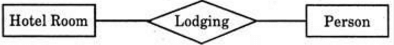If we wish to store information about the rent payment to be made by person (s) occupying different hotel rooms, then this information should appear as an attribute of

Detailed Solution for Test: Normal Forms- 1 - Question 15

Lodging is the only attribute relating person and hotel room.

Test: Normal Forms- 1 - Question 16

A table has fields Fl, F2, F3, F4, F5 with the following functional dependencies   F1 → F3   F2→ F4   (F1 . F2) → F5 In terms of Normalization, this table is in

Detailed Solution for Test: Normal Forms- 1 - Question 16

First Normal Form A relation is in first normal form if every attribute in that relation is singled valued attribute. Second Normal Form A relation is in 2NF iff it has No Partial Dependency, i.e.no non-prime attribute (attributes which are not part of any candidate key) is dependent on any proper subset of any candidate key of the table. This table has Partial Dependency f1->f3, f2-> f4 given (F1,F2) is Key So answer is A

Test: Normal Forms- 1 - Question 17

Which of the following is NOT a superkey in a relational schema with attributes V, W, X, Y, Z and primary key V Y ?

Detailed Solution for Test: Normal Forms- 1 - Question 17

Super key = Candidate Key + other attributes. But option B does not include Y which is a part of PK or candidate key.

Test: Normal Forms- 1 - Question 18

Let R (A, B, C, D, E, P, G) be a relational schema in which the following functional depen­dencies are known to hold: AB → CD, DE → P, C → E, P → C and B → G. The relational schema R is

Detailed Solution for Test: Normal Forms- 1 - Question 18

Candidate key = AB
B->G is partial dependency
So, not in 2NF

Test: Normal Forms- 1 - Question 19

Which option is true about the SQL query given below?

SELECT firstName, lastName
FROM Employee
WHERE lastName BETWEEN 'A%' AND 'D%';

Detailed Solution for Test: Normal Forms- 1 - Question 19

The BETWEEN operator works with the range of character values also.

Test: Normal Forms- 1 - Question 20

Which of the given options define a transaction correctly?

Detailed Solution for Test: Normal Forms- 1 - Question 20

A database transaction consists of one or more DML statements to constitute one consistent change in data, or a DDL statement or a DCL command (GRANT or REVOKE). It starts with the first DML statement and ends with a DCL or DDL or TCL (COMMIT or ROLLBACK) command. Note that DDL and DCL commands hold auto commit feature.

## GATE Computer Science Engineering(CSE) 2024 Mock Test Series

151 docs|216 tests
Information about Test: Normal Forms- 1 Page
In this test you can find the Exam questions for Test: Normal Forms- 1 solved & explained in the simplest way possible. Besides giving Questions and answers for Test: Normal Forms- 1, EduRev gives you an ample number of Online tests for practice

## GATE Computer Science Engineering(CSE) 2024 Mock Test Series

151 docs|216 tests